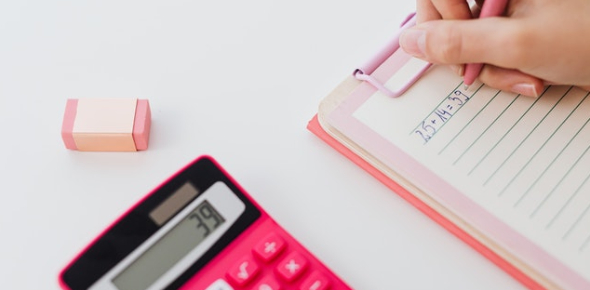# General Maths Quiz Questions And Answers

20 Questions | Attempts: 3815
ShareSettingsHow good is your general Maths? Try to attempt these questions and answers from this quiz and check where you lack and need more practice? General math is a major branch of basic mathematics that includes all math operations like subtraction, addition, multiplication, and division. Fractions, percentages, ratios, and proportions are also covered in this field. So, without further ado, let's start the quiz below. Evaluate yourself today and see how you score.

• 1.
What do you call the answer in Subtraction?
• A.

Difference

• B.

Plus

• C.

Sum

• D.

None

• 2.
What are the missing numbers in the sequence? 2840 2940 _____ ____ 3,240 3,340
• A.

2,941 3,141

• B.

3.040 3,140

• C.

3,000 3,241

• D.

2,090 2,990

• 3.
What is the sign used in subtraction?
• A.

Plus

• B.

Minus

• C.

• D.

Sum

• 4.
Look at these patterns. What are the next two numbers? 8,454      8,458 ____ 8,466 _________
• A.

8,476    8,477

• B.

8,460     8,600

• C.

8,462     8,470

• D.

8,462      8,466

• 5.
• A.

Difference

• B.

Sum

• C.

Minus

• D.

Take away

• 6.
What is the sum of 9,684 and 4,998?
• A.

14,681

• B.

14,682

• C.

14,683

• D.

14,684

• 7.
• A.

Minus

• B.

Plus

• C.

Subtract

• D.

Take away

• 8.
Is 8,070 an even or odd number?
• A.

Odd

• B.

Even

• C.

Both

• D.

None

• 9.
What is the difference between 4,938 and 5,001?
• A.

53

• B.

43

• C.

73

• D.

63

• 10.
What is the missing number? _________8,123   8,223     8,323
• A.

8,120

• B.

8,023

• C.

8,134

• D.

8,022

• 11.
What is 10 more than 8,005?
• A.

8,125

• B.

8,025

• C.

8,015

• D.

8,035

• 12.
What is the missing number in 7,483 - ______= 2,761?
• A.

4,722

• B.

4,723

• C.

4,724

• D.

4,725

• 13.
How much less 3,846 than 6,012?
• A.

2,167

• B.

2,166

• C.

2,168

• D.

2,169

• 14.
What is 100 less than 9,182?
• A.

9,282

• B.

9,382

• C.

9,082

• D.

9,472

• 15.
.In these numbers : 2,326   3,581    1,229     5,944    4,127 What is the total of all odd numbers?
• A.

8,932

• B.

8,935

• C.

8,937

• D.

8,938

• 16.
In these numbers : 2,326      3,581      1,229       5,944         4,127 What is the difference between two even numbers?
• A.

3,628

• B.

3,617

• C.

3,619

• D.

3,618

• 17.
In these numbers : 2,326       3,581       1,229       5,944        4,127 Add together the two smallest numbers?
• A.

3,556

• B.

3,557

• C.

3,550

• D.

3,555

• 18.
On the first day of the week, Pat had 139 stickers and she got 22 more on the second day. How many stickers does she have altogether?
• A.

147 stickers

• B.

151 stickers

• C.

161 stickers

• D.

117 stickers

• 19.
19-20. Ms. Sam is the teacher for Grade 3. She has 39 boys and 29 girls in her class. How many students are there in the class?
• A.

68 boys

• B.

68 girls

• C.

71 students

• D.

68 students

• 20.
How many more boys than girls?
• A.

10 boys

• B.

10 girls

• C.

10 students

• D.

10 teachers

## Related TopicsBack to top
×

Wait!
Here's an interesting quiz for you.